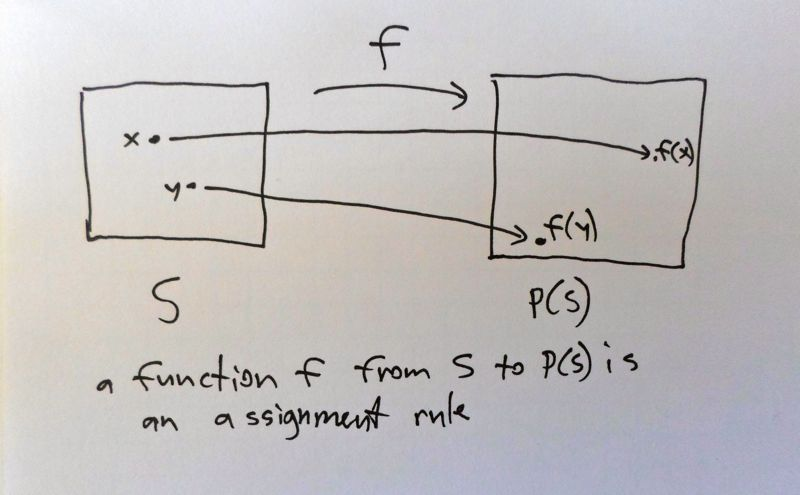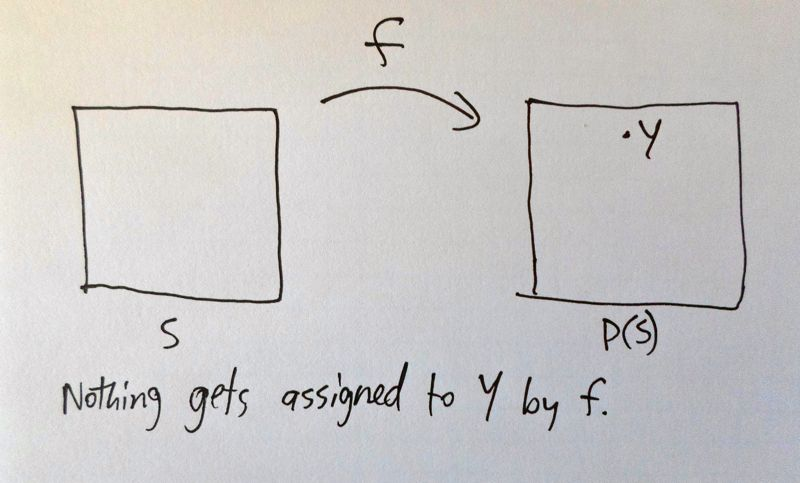# In-Depth

We will prove that, for every set $$\mathscr{S}$$, $$| P(\mathscr{S})| > |\mathscr{S}|$$.

Let $$\mathscr{S}$$ be a set, finite or infinite. By definition, $$P(\mathscr{S})$$, called the power set, is the set of subsets of $$\mathscr{S}$$. For example, if $$\mathscr{S} = \{a, b, c\}$$, then

$P(\mathscr{S}) = \{ \{a\}, \{b\}, \{c\}, \{a,b\}, \{a,c\}, \{b,c\}, \{ \}, \{a,b,c\} \}.$

Some of the subsets of $$\mathscr{S}$$ are singleton subsets, those with one element. In this example, they are $$\{a\}$$, $$\{b\}$$, and $$\{c\}$$. The collection of singleton subsets of $$\mathscr{S}$$, taken together, form a subset of $$P(\mathscr{S})$$.

We can make a one-to-one correspondence between $$\mathscr{S}$$ and the set of singleton subsets of $$\mathscr{S}$$; each element $$s$$ of $$\mathscr{S}$$ gets assigned to the singleton $$\{s\}$$. This is a valid one-to-one correspondence, between $$\mathscr{S}$$ and a subset of $$P(\mathscr{S})$$. So we know that $$\mathscr{S}$$ has to be no larger than $$P(\mathscr{S})$$.

Next we will show that $$P(\mathscr{S})$$ is not the same size as $$\mathscr{S}$$, and this will be enough to conclude that $$| P(\mathscr{S})| > |\mathscr{S}|$$.

To show $$\mathscr{S}$$ and $$P(\mathscr{S})$$ are not the same size, we must show that there can be no one-to-one correspondence between them. Here it's helpful to think of assignments between sets as functions.

A function $$f$$ from $$\mathscr{S}$$ to $$P(\mathscr{S})$$ is an assignment rule. For each element $$x$$ in $$\mathscr{S}$$, it assigns an element $$f(x)$$ in $$P(\mathscr{S})$$. As far as we're concerned here, that is all a function is -- an assignment rule. But in this case, since $$P(\mathscr{S})$$ is the set of subsets of $$\mathscr{S}$$, a function from $$\mathscr{S}$$ to $$P(\mathscr{S})$$ seems a little bizarre. To each element $$x$$ in $$\mathscr{S}$$, the function $$f$$ assigns a subset $$f(x)$$ of $$\mathscr{S}$$.A one-to-one correspondence is a special type of function; it's a function that makes sure everything gets hit once and only once. In other words, a function $$f$$ from $$\mathscr{S}$$ to $$P(\mathscr{S})$$ is a one-to-one correspondence if every element in $$P(\mathscr{S})$$ gets hit exactly once by $$f$$. More precisely, $$f$$ is a one-to-one correspondence if, for every subset $$Z$$ of $$\mathscr{S}$$ -- i.e. every element $$Z$$ of $$P(\mathscr{S})$$ -- there is one and exactly one element $$t$$ in $$\mathscr{S}$$, so that $$f(t) = Z$$.

What we will show is that any function $$f$$ from $$\mathscr{S}$$ to $$P(\mathscr{S})$$ fails to hit everything. In other words, given a function $$f$$, we'll show that we can construct a special subset $$Y$$ of $$\mathscr{S}$$ in such a way that we are sure no element of $$\mathscr{S}$$ gets assigned to $$Y$$ by $$f$$.

So now suppose we have a function $$f$$ from $$\mathscr{S}$$ to $$P(\mathscr{S})$$. Consider the following subset of $$\mathscr{S}$$, i.e. element of $$P(\mathscr{S})$$.

$$Y= \{$$ elements $$s$$ in $$\mathscr{S}$$, such that $$s$$ is not an element of $$f(s) \}$$

This may seem like an odd way to define a subset, describing it in words. But it is perfectly acceptable (at least in this basic version of set theory we are using). The words clearly give a condition for deciding what elements of $$\mathscr{S}$$ should be included in $$Y$$. Mathematicians do this all the time. For example, we could describe the set of even natural numbers as

$$2\mathbb{N} = \{$$ all natural numbers that are divisible by two$$\}$$.

What are the elements of $$Y$$? Each element $$s$$ in $$\mathscr{S}$$ is getting assigned to some element of $$P(\mathscr{S})$$, by the function $$f$$. This $$f(s)$$ is a subset of $$\mathscr{S}$$, so it makes sense to ask, is $$s$$ an element of $$f(s)$$? If $$s$$ is not an element of $$f(s)$$, then we put it into $$Y$$. If $$s$$ is an element of $$f(s)$$, then it doesn't meet the criterion and doesn't belong in $$Y$$. We can run through this for every element of $$\mathscr{S}$$, and in the end we get $$Y$$.

Now comes the clever part. Remember what we just said: for each element $$s$$ in $$\mathscr{S}$$, if $$s$$ is not an element of $$f(s)$$, then we put it into $$Y$$. But in this case, $$s$$ is an element of $$Y$$ but not an element of $$f(s)$$; in other words, $$Y$$ has something $$f(s)$$ doesn't have. So $$Y$$ and $$f(s)$$ can't be the same set. On the other hand, if $$s$$ is an element of $$f(s)$$, then it doesn't meet the criterion and doesn't go into $$Y$$. In this case, $$f(s)$$ has something that $$Y$$ doesn't have, so $$f(s)$$ and $$Y$$ cannot be the same set. So whether or not $$s$$ was in $$f(s)$$ or not in $$f(s)$$, we concluded that $$f(s)$$ and $$Y$$ were not the same set.

What did we just conclude? For each element $$s$$ in $$\mathscr{S}$$, the sets $$f(s)$$ and $$Y$$ are not the same. Now, thinking of $$f$$ as a function from $$\mathscr{S}$$ to $$P(\mathscr{S})$$, this says that there is no element of $$\mathscr{S}$$ getting assigned to $$Y$$! The set $$Y$$ is the special element of $$P(\mathscr{S})$$ that is not -- cannot -- be hit by the function $$f$$.This trick works for any function from $$\mathscr{S}$$ to $$P(\mathscr{S})$$. We can always construct a special element of $$P(\mathscr{S})$$ that does not get hit by the function. The conclusion is that any function from $$\mathscr{S}$$ to $$P(\mathscr{S})$$ will fail to be a one-to-one correspondence; there can be no one-to-one correspondence between $$\mathscr{S}$$ and $$P(\mathscr{S})$$; and so $$\mathscr{S}$$ is strictly smaller than $$P(\mathscr{S})$$.

Top